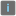• # how do i solve this

## Question related to missionMultiply (Intro)

im not sure what the task is asking of me im to change the variables in the code but it looks like its already solved

def mult_two(a, b): # your code here return None

if name == 'main': print("Example:") print(mult_two(3, 2))

```# These "asserts" are used for self-checking and not for an auto-testing
assert mult_two(3, 2) == 6
assert mult_two(1, 0) == 0
print("Coding complete? Click 'Check' to earn cool rewards!")
```

what code am I supposed to write under def mult_two I dont understand3